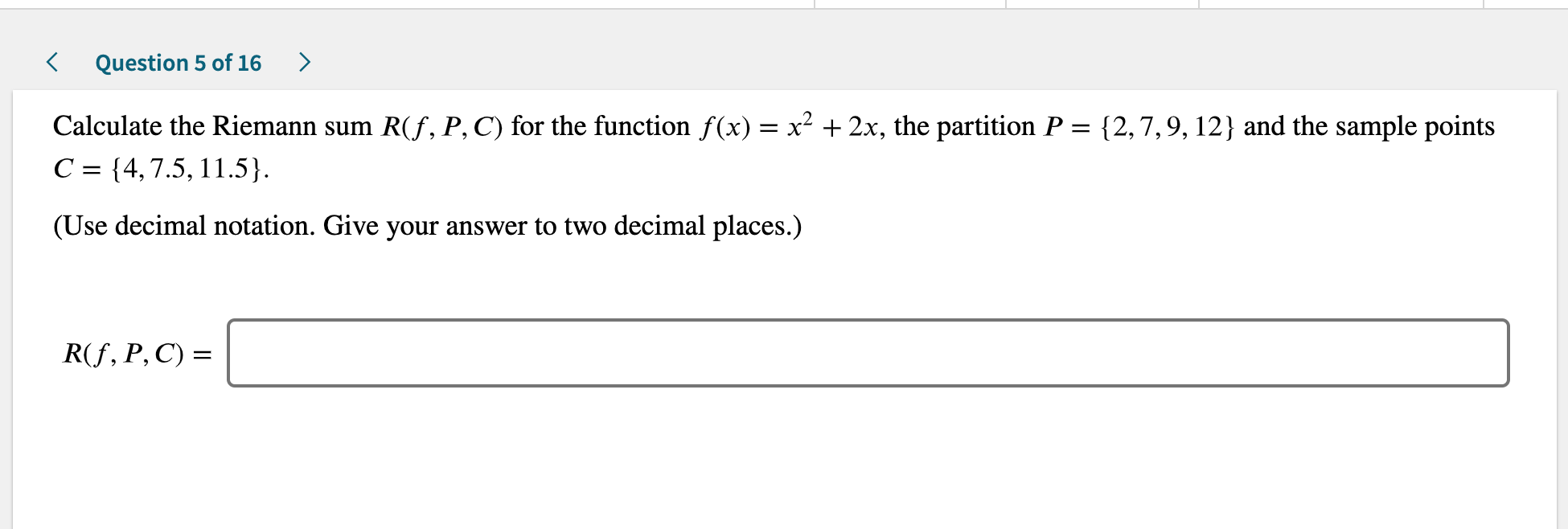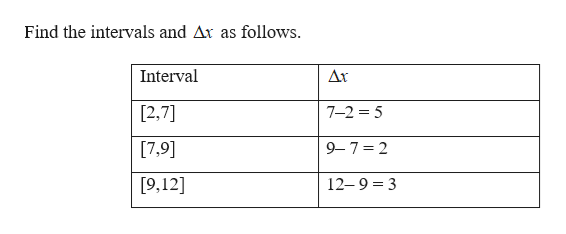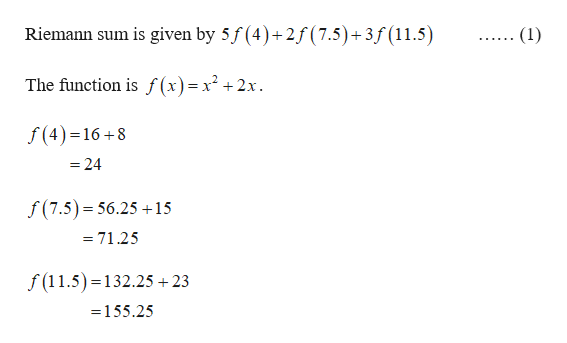# Question 5 of 16Calculate the Riemann sum R(f, P, C) for the function f(x) x2 +2x, the partition P = {2,7,9,12} and the sample pointsC {4,7.5, 11.5}(Use decimal notation. Give your answer to two decimal places.)R(f, P, C)

Question
12 viewshelp_outlineImage TranscriptioncloseQuestion 5 of 16 Calculate the Riemann sum R(f, P, C) for the function f(x) x2 +2x, the partition P = {2,7,9,12} and the sample points C {4,7.5, 11.5} (Use decimal notation. Give your answer to two decimal places.) R(f, P, C) fullscreen
check_circle

Step 1

The given partition points are {2, 7, 9, 12} and sample points {4, 7.5, and 11.5}.help_outlineImage TranscriptioncloseFind the intervals and Ar as follows Дr Interval [2,7] 7-2 5 [7,9] 9-7 2 12-9 3 9,12] fullscreen
Step 2help_outlineImage TranscriptioncloseRiemann sum is given by 5f (4)+2f(7.5) + 3f (11.5) (1) The function is f(x)= x2+2x. f(4) 16+8 -24 f (7.5) 56.25+15 =71.25 f(11.5) 132.25 +23 =155.25 fullscreen

### Want to see the full answer?

See Solution

#### Want to see this answer and more?

Solutions are written by subject experts who are available 24/7. Questions are typically answered within 1 hour.*

See Solution
*Response times may vary by subject and question.
Tagged in